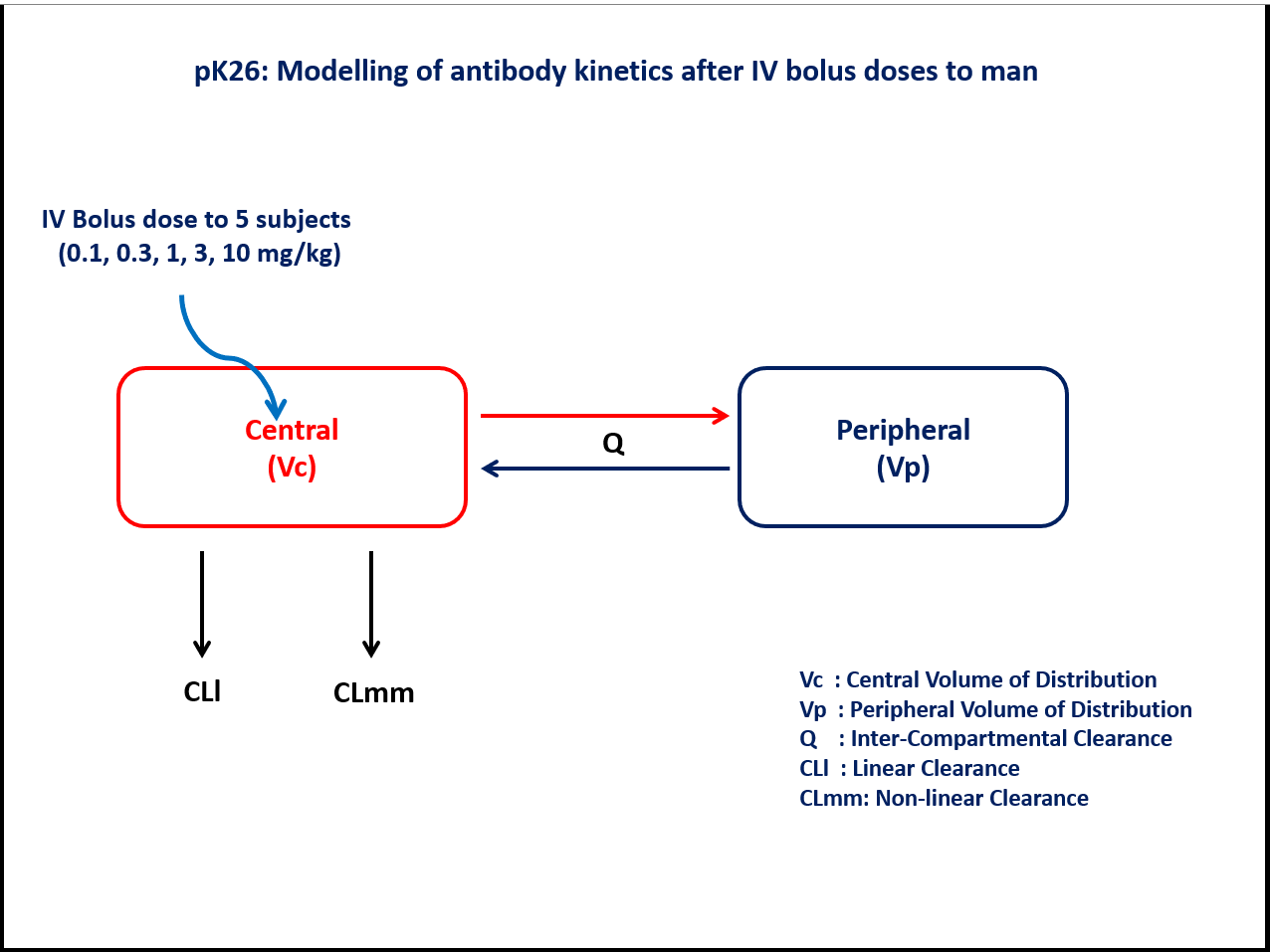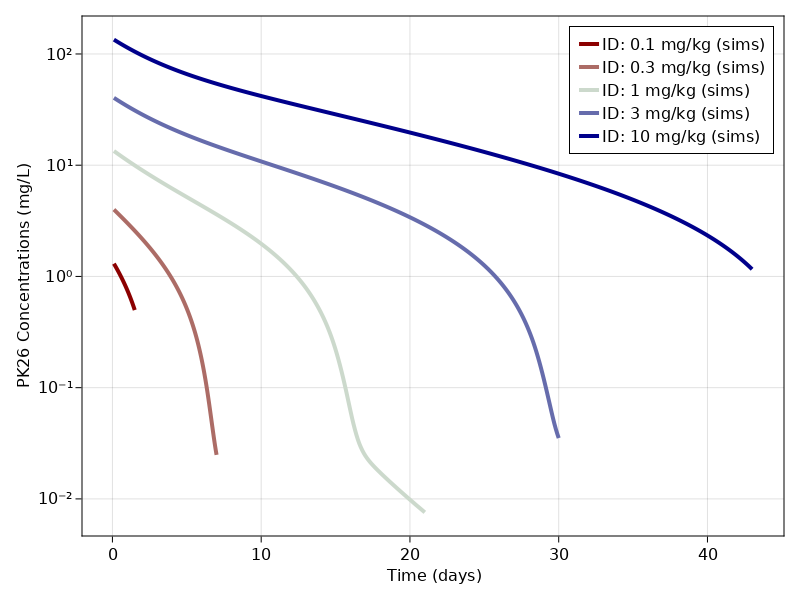# Exercise PK26 - Modeling of antibody kinetics after IV doses to man

### Background

Following info:

• Structural model - Two compartment model with parallel linear and Non-linear elimination

• Route of administration - IV bolus (Single dose)

• Dosage Regimen - 0.1mg/kg, 0.3mg/kg, 1mg/kg, 3mg/kg, and 10 mg/kg

• Number of Subjects - 5### Learning Outcomes

To understand the antibody kinetics with linear and nonlinear elimination after IV bolus dose in man.

### Objectives

• To build a two compartment model with parallel linear and non-linear elimination to understand the antibody kinetics.

• To simulate 5 subjects after single dose IV bolus administration

### Libraries

Call the "necessary" libraries to get started.

using Random
using Pumas
using PumasUtilities
using CairoMakie


### Model

Two compartment model with parallel linear and Non- linear elimination

pk_26            = @model begin
desc        = "Parallel Linear and Non-linear Elimination Model"
timeu       = u"hr"
end

@param begin
"Maximum rate of Metabolism(mg/hr/kg)"
tvvmax      ∈ RealDomain(lower=0)
"Michaelis constant (mg/L/kg)"
tvkm        ∈ RealDomain(lower=0)
"Volume of Peripheral Compartment(L/kg)"
tvvp        ∈ RealDomain(lower=0)
"Volume of Central Compartment(L/kg)"
tvvc        ∈ RealDomain(lower=0)
"Inter-compartmental Clearance(L/hr/kg)"
tvq         ∈ RealDomain(lower=0)
"Linear Clearance(L/hr/kg)"
tvcll       ∈ RealDomain(lower=0)
Ω           ∈ PDiagDomain(6)
"Proportional RUV"
σ²_prop     ∈ RealDomain(lower=0)
end

@random begin
η           ~ MvNormal(Ω)
end

@pre begin
Vmax        = tvvmax*exp(η)
Km          = tvkm*exp(η)
Vp          = tvvp*exp(η)
Vc          = tvvc*exp(η)
Q           = tvq*exp(η)
CLl         = tvcll*exp(η) # Linear clearance
# CLmm       = Vmax/(Km+C)     Non-linear clearance
end

@dynamics begin
Central'    = -(Vmax/(Km+(Central/Vc)))*(Central/Vc) - CLl*(Central/Vc)-(Q/Vc)*Central +(Q/Vp)*Peripheral
Peripheral' = (Q/Vc)*Central -(Q/Vp)*Peripheral
end

@derived begin
cp          = @. Central/Vc
"""
Observed Concentration (mg/L)
"""
dv          ~ @. Normal(cp, sqrt(cp^2*σ²_prop))
end
end

PumasModel
Parameters: tvvmax, tvkm, tvvp, tvvc, tvq, tvcll, Ω, σ²_prop
Random effects: η
Covariates:
Dynamical variables: Central, Peripheral
Derived: cp, dv
Observed: cp, dv


### Parameters

The parameters are as given below. tv represents the typical value for parameters.

• $Vmax$ - Maximum rate of Metabolism(mg/hr/kg)

• $Km$ - Michaelis constant (mg/L/kg)

• $Vp$ - Volume of Peripheral Compartment(L/kg)

• $Vc$ - Volume of Central Compartment(L/kg)

• $Q$ - Inter-compartmental Clearance(L/hr/kg)

• $CLl$ - Linear Clearance(L/hr/kg)

• $Ω$ - Between Subject Variability

• $σ²_prop$ - Residual error

param = ( tvvmax  = 0.0338,
tvkm    = 0.0760,
tvvp    = 0.0293,
tvvc    = 0.0729,
tvq     = 0.0070,
tvcll   = 0.0069,
Ω       = Diagonal([0.0,0.0,0.0,0.0,0.0,0.0]),
σ²_prop = 0.04)

(tvvmax = 0.0338, tvkm = 0.076, tvvp = 0.0293, tvvc = 0.0729, tvq = 0.007,
tvcll = 0.0069, Ω = [0.0 0.0 … 0.0 0.0; 0.0 0.0 … 0.0 0.0; … ; 0.0 0.0 … 0.
0 0.0; 0.0 0.0 … 0.0 0.0], σ²_prop = 0.04)


### Dosage Regimen

5 subjects received an IV bolus dose of 0.1, 0.3, 1, 3 and 10 mg/kg respectively at time=0

DR1 = DosageRegimen(0.1, time = 0)
s1  = Subject(id = "0.1 mg/kg", events = DR1, time = 0.1:0.01: 1.5)
DR2 = DosageRegimen(0.3, time = 0)
s2  = Subject(id = "0.3 mg/kg", events = DR2, time = 0.1:0.01:7)
DR3 = DosageRegimen(1, time = 0)
s3  = Subject(id = "1 mg/kg", events = DR3, time = 0.1:0.1:21)
DR4 = DosageRegimen(3, time = 0)
s4  = Subject(id = "3 mg/kg", events = DR4, time = 0.1:0.1:30)
DR5 = DosageRegimen(10, time = 0)
s5  = Subject(id = "10 mg/kg", events = DR5, time = 0.1: 0.1:43)

pop = [s1,s2,s3,s4,s5]

Population
Subjects: 5
Observations:


### Simulation

To simulate plasma concentration data for 5 subjects with specific obstimes.

Random.seed!(123)
sim = simobs(pk_26, pop, param)


### Visualization

f, a, p = sim_plot(pk_26, sim,
observations = :cp,
color = :redsblues,
linewidth = 4,
axis = (xlabel = "Time (days)",
ylabel = "PK26 Concentrations (mg/L)",
xticks = 0:10:40, yscale = log10))
axislegend(a)
f